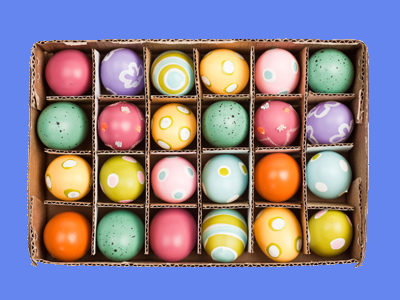6 x 4 = 24 and 4 x 6 = 24 as well!

# Year 2 Calculation - Multiplication in any Order

## Enjoyable Quiz for Children Aged 5 to 7

This quiz addresses the requirements of the National Curriculum KS1 Maths and Numeracy for children aged 6 and 7 in year 2. Specifically this quiz is aimed at the section dealing with recognising that the multiplication of two numbers can be done in any order.

By Year 2, children should be able to recognise the commutative property of a multiplication calculation. This means that the two numbers being multiplied can be done in either order, and still give the same answer. For example, 5 x 4 gives the same result as 4 x 5. They may use this skill to answer any questions involving times tables they are unfamiliar with (if they don't know 5 x 7, they may know 7 x 5 for instance). Cumulative property also applies when 3, 4 or any other group of numbers are to be multiplied. The calculation can be done in any order and the answer will be the same.

Question 1
I don't know my 7 times table yet. How could I work out 5 x 7?
By quickly learning the 7 times table
By doing 7 x 5 instead
By adding 7 and 5 together
5 x 7 = 35 and 7 x 5 is 35 too
Question 2
Which of the following pairs give an answer of 60?
60 x 2 and 2 x 60
30 x 2 and 2 x 30
15 x 3 and 3 x 15
40 x 4 and 4 x 40
2 x 30 gives an answer of 60, as does 30 x 2
Question 3
Which number is missing?
20 x ? = 80
60
40
24
4
4 x 20 = 80 and 20 x 4 = 80 too
Question 4
When you multiply two numbers...
you can multiply them in any order
you will get an answer of more than 50
you will also find the difference
When multiplying two numbers, it doesn't matter which way round they go, you will still get the same answer
Question 5
What could make this calculation easier to work out?
5 x 12
Quickly learning the 12 times table
Changing the numbers around to 12 x 5
Finding the difference between the two numbers
5 x 12 = 60 and 12 x 5 also gives the same answer
Question 6
What is 100 x 4?
410
40
104
400
Changing the order of the numbers makes this 4 x 100, which is easier to work out
Question 7
What is 50 x 2?
52
100
25
70
2 x 50 is much easier to work out!
Question 8
Which of the following pairs give an answer of 20?
20 x 2 and 2 x 20
4 x 5 and 5 x 4
10 x 4 and 4 x 10
10 x 20 and 20 x 10
4 x 5 = 20, as does 5 x 4
Question 9
Which number is missing?
? x 8 = 16
4
24
12
2
If you know that 8 x 2 = 16, then 2 x 8 = 16 also
Question 10
5 x 6 gives the same answer as...
40 x 5
4 x 50
5 + 4
6 x 5
They both give an answer of 30